## Surface tension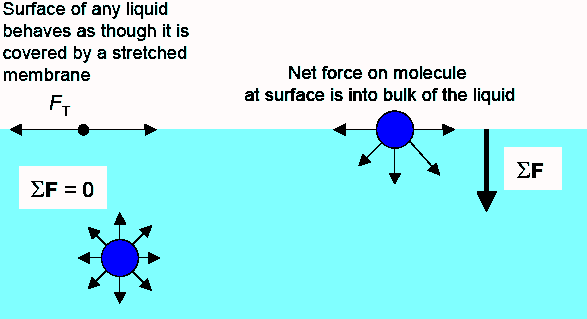The surface of a liquid behaves as though it is covered by a stretched membrane.  The strength of the membrane depends on the liquid.  It is, for example, much higher for pure water than for soapy water.  Cohesive forces that act between the molecules of the liquid without chemical bonding give rise to the surface tension.  [Molecules in liquids experience strong intermolecular attractive forces.  When those forces act between like molecules, they are referred to as cohesive forces.  When the attractive forces act between unlike molecules, they are said to be adhesive forces.]

The molecules at the surface do not have other neighbors of the same kind on all sides.  They experience a net attractive force toward the bulk of the liquid.  This force results in a surface "film" which makes it more difficult to move an object through the surface than to move it, when it is completely submersed.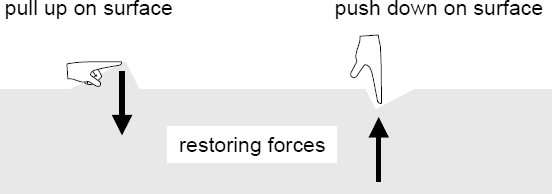The surface of a liquid behaves like an elastic membrane.  A surface molecule which is displaced by a small amount is acted on by a restoring force due to cohesive forces.

If there are no competing external forces present, a liquid system will minimize its surface area.  Soap bubbles and raindrops are spherical because the sphere is the shape with the smallest surface to volume ratio.

Liquid Surface Tension γ (N/m)
water (20 oC) 0.073
water (100 oC) 0.059
soapy water (20 oC) 0.025
alcohol 0.022
glycerine 0.063
mercury 0.513

The surface tension γ is defined as the force along a line of unit length.  The force is parallel to the surface and perpendicular to the line.  Surface tension is therefore measured in units of N/m in SI units.  The surface tension γ for different surfaces is listed in the table on the right.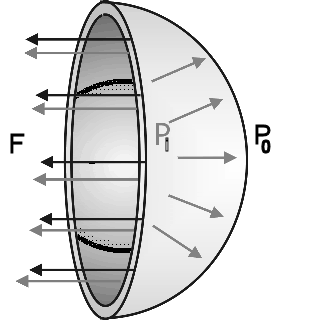Picture a soap bubble.  The surface tension balances the outward force due to the pressure difference between the inside and the outside air.  We have two surfaces, the inner and the outer surface of the bubble.  The force from surface tension is F = 2γL = 2γ2πr = 4γπr.  For the bubble to be stable and not collapse, the pressure inside the bubble must be higher than the pressure on the outside.  The force do to the pressure difference must balance the force from the surface tension. The force to the pressure difference is (Pi - Po)πr2.

For a single spherical surface balance is achieved if Pi - Po = 2γ/r.  This is known as Laplace's law for a spherical membrane.
For a soap bubble with two surfaces Pi - Po = 4γ/r.

#### Problem:

Calculate the gauge pressure inside a soap bubble 2 cm in radius using the surface tension for soapy water γ = 0.025 N/m.

Solution:

• Reasoning:
Use Laplace's law for a spherical membrane.  For a soap bubble with two surfaces Pi - Po = 4γ/r.
• Details of the calculation:
Pi - Po = 4γ/r.  Po = atmospheric pressure, so Pi - Po = gauge pressure.
Pi - Po = (4*0.025 N/m)/(0.02 m) = 5 Pa.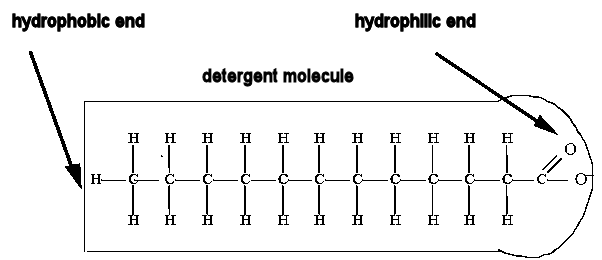Detergents lower the surface tension of water.  Detergent molecules have two ends, one hydrophobic end, repelled by water and one hydrophilic end, attracted to water.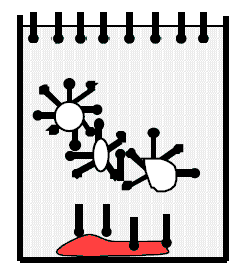In water detergent molecules on the surface are aligned with their hydrophobic ends away from the surface.  They break the surface and thus lower the surface tension.  Other detergent molecules are dispersed throughout the water.  Particles of organic matter are rendered soluble by being coated with these detergent molecules.  The hydrophobic ends stick to the particles and the hydrophilic ends point outwards.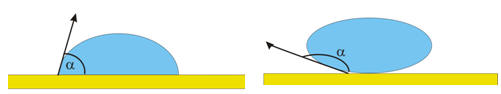Wetting is the ability of liquids to form interfaces with solid surfaces.  The contact angle θ between the liquid and the solid surface is a measure of the degree of wetting.  A smaller the contact angle and a smaller the surface tension result in a greater the degree of wetting.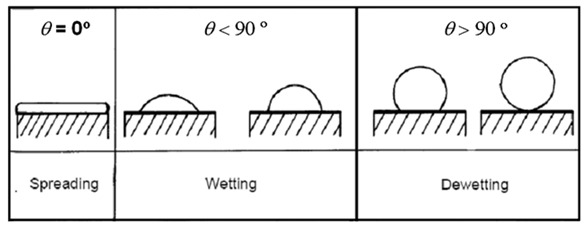When adhesive forces are greater than cohesive forces, the contact angle θ lies between 0 and 90o.  When adhesive forces are smaller than cohesive forces, the contact angle θ lies between 90o and 180o.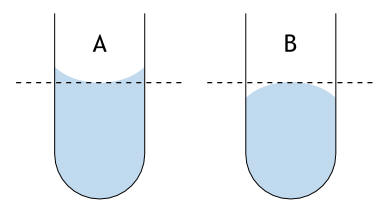(A)  A concave meniscus  (example: water in glass)
(B)  A convex meniscus  (example: mercury in glass)

For liquid standing in a container, a meniscus forms.  It can be convex or concave.  A concave meniscus indicated that the molecules of the liquid have a stronger attraction to the material of the container (adhesion) than to each other (cohesion).  A convex meniscus indicates the molecules have a stronger attraction to each other than to the material of the container.mg = (F/L) cosθ 2πr (net force = 0)

ρπr2h g = γ cosθ 2πr (solve for h)

Adhesion and surface tension together can produce capillary action.  Capillary action occurs when the adhesion to the walls is stronger than the cohesive forces between the liquid molecules.  Adhesion of water to the walls of a vessel results in an upward force on the liquid at the edges.  The surface tension keeps the surface intact, so the whole liquid surface is dragged upward.

The maximum height to which the liquid will rise through capillary action is given by

h = 2γcosθ/(ρgr).

Then the weight of the risen liquid is balanced by the surface tension.
(See diagram on the right.)